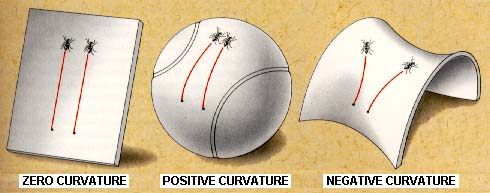# Differential GeometryFrom "A Journey into Gravity and Spacetime" by Wheeler.

### Course Info

 Instructor: Facundo Mémoli,   m e m o l i @ m a t h . o s u . e d u Course code: Math 6702 -- Spring 2018 Times: MWF 1.50--2.45 Location: TBA. Topics: Riemannian manifolds, distance, volume; covariant derivatives, Levi-Civita connection; geodesics, curvatures: sectional, Ricci, scalar; Jacobi fields, first and second variation of theenergy functional, conjugate points, Rauch comparison, Hopf-Rinow, Hadamard, Bishop and Bishop-Gromov theorems. Other topics: Spectral geometry, families of manifolds with bounded geometry. Assessment: TBA. Prerequisites: Some degree of familiarity with differentiable manifolds and differential geometry of curves and surfaces. See below for required background knowledge.

### Lectures and resources

 Assumed background. To read and digest on your own before the first day of class: Chapter 1 of [GHL] and Chapters 1 to 4 of Do Carmo's Differential Geometry of Curves and Surfaces. Books: The book by Gallot, Hulin & LaFontaine ([GHL] from now on) is a good resource. I will also use parts of Sakai's book ([S] from now on), and Petersen's book ([P] from now on). The book by Berger "A panoramic view of Riemannian Geometry" ([B]) is a very good read. The following paper by Petersen is a very good summary of some global results in RG: "Aspects of global Riemannian Geometry" ([AGRG]). Lecture 0 (M Jan. 8th). Welcome, contents, syllabus, organization. Lecture 1 (W Jan. 10th). $(M,g^M)\in\mathcal{R}$ and maps $F:M\rightarrow N$ between RMs. Metric tensors in \mathbb{R}^n. Riemannian metrics on manifolds. Existence of a Riemannian metric. Some examples. Lecture 2 (F Jan 12th). Length of curves. RMs as metric spaces.